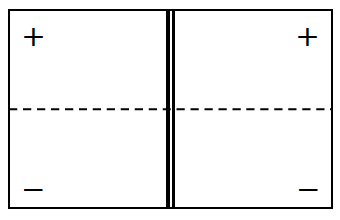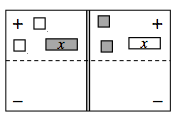### Home > CC3 > Chapter 3 > Lesson 3.1.4 > Problem3-38

3-38.

For the following equations, draw a picture of the tiles on an Equation Mat like the one shown at right. Then use “legal” moves to simplify and solve for the variable. Record your work.1. $−2+x=−x+2$1. $2+3x=x+7$

$x=2.5$

Use the eTools below to help solve the problems.
Click the link at right for the full version of the eTool: 3-38 HW eTool (CPM)